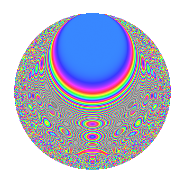# Properties

 Label 693.2.bdLevel 693 Weight 2 Character orbit bd Rep. character $$\chi_{693}(419,\cdot)$$ Character field $$\Q(\zeta_{6})$$ Dimension 160 Newform subspaces 1 Sturm bound 192 Trace bound 0

# Related objects

## Defining parameters

 Level: $$N$$ = $$693 = 3^{2} \cdot 7 \cdot 11$$ Weight: $$k$$ = $$2$$ Character orbit: $$[\chi]$$ = 693.bd (of order $$6$$ and degree $$2$$) Character conductor: $$\operatorname{cond}(\chi)$$ = $$63$$ Character field: $$\Q(\zeta_{6})$$ Newform subspaces: $$1$$ Sturm bound: $$192$$ Trace bound: $$0$$

## Dimensions

The following table gives the dimensions of various subspaces of $$M_{2}(693, [\chi])$$.

Total New Old
Modular forms 200 160 40
Cusp forms 184 160 24
Eisenstein series 16 0 16

## Trace form

 $$160q + 80q^{4} + 2q^{7} + 8q^{9} + O(q^{10})$$ $$160q + 80q^{4} + 2q^{7} + 8q^{9} + 18q^{14} - 4q^{15} - 80q^{16} + 12q^{18} + 28q^{21} - 24q^{23} - 80q^{25} + 16q^{28} - 72q^{29} - 68q^{30} + 60q^{32} - 12q^{36} - 8q^{37} - 16q^{39} - 30q^{42} + 16q^{43} + 48q^{46} - 14q^{49} + 24q^{50} + 8q^{51} + 24q^{56} + 64q^{57} + 32q^{60} - 60q^{63} - 160q^{64} + 156q^{65} - 28q^{67} + 12q^{70} + 108q^{72} - 12q^{74} + 28q^{78} - 4q^{79} - 104q^{81} + 98q^{84} - 180q^{86} + 12q^{91} - 12q^{92} - 132q^{93} - 144q^{95} + O(q^{100})$$

## Decomposition of $$S_{2}^{\mathrm{new}}(693, [\chi])$$ into newform subspaces

Label Dim. $$A$$ Field CM Traces $q$-expansion
$$a_2$$ $$a_3$$ $$a_5$$ $$a_7$$
693.2.bd.a $$160$$ $$5.534$$ None $$0$$ $$0$$ $$0$$ $$2$$

## Decomposition of $$S_{2}^{\mathrm{old}}(693, [\chi])$$ into lower level spaces

$$S_{2}^{\mathrm{old}}(693, [\chi]) \cong$$ $$S_{2}^{\mathrm{new}}(63, [\chi])$$$$^{\oplus 2}$$

## Hecke Characteristic Polynomials

There are no characteristic polynomials of Hecke operators in the database